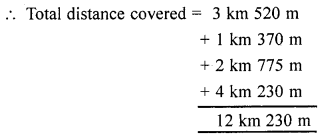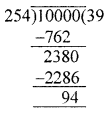## ML Aggarwal Class 6 Solutions for ICSE Maths Model Question Paper 1

Choose the correct answer from the given four options (1-2):
Question 1.
If the sum of two integers is -21 and one of them is -10 then the other is
(a) -32
(b) 32
(c) -11
(d) 11
Solution:
Let the other integer be x
∴ x + (-10) = -21
x = -21 + 10
x = -11 (c)

Question 2.
The number of natural numbers between the smallest natural number and the greatest 2-digit number is
(a) 90
(b) 97
(c) 98
(d) 99
Solution:
Smallest natural number = 1
Greatest 2-digit number = 99
⇒ So the natural numbers between 1 and 99 are
2, 3, 4, ………….., 98
∴ Total Natural numbers = 98 – 1 = 97

Question 3.
Find the value of 25 × 37 × 8 × 6 by suitable arrangement.
Solution:
25 × 37 × 8 × 6
= 25 × 8 × 37 × 6
= 200 × 222
= 44400

Question 4.
Write four consecutive integers preceding -97.
Solution:
The four consecutive integers preceding
-97 is
= -97 – 1 = -98
= -98 – 1 = -99
= -99 – 1 = -100
= -100 – 1 = -101

Question 5.
Write the greatest and the smallest 4-digit numbers using four different digits with the condition that 5 occurs at ten’s place.
Solution:
Greatest 4-digit number when digit 5 always at tens place = 9857
Smallest 4-digit number when digit 5 is a tens place = 1052

Question 6.
Write all possible natural numbers formed by the digits 7, 0 and 3. Repetition of digits is not allowed.
Solution:
The given digits are 7, 0, 3 and repetition of digits is not allowed.
The one-digit numbers that can be formed are 7 and 3.
We are required to write 2-digit numbers.
Out of the given digits, the possible ways of choosing the two digits are
7, 0, 3, 0, 3, 7
Using the digits 7 and 0, the numbers are 70.
Similarily, Using the digits 3 and 0, the numbers are 30
Using the digits 3 and 7, the numbers are 37 and 73.
Hence, all possible 2-digit numbers are 30, 70, 37, 73
Now, We are required to write 3-digit numbers using the digits 7, 0, 3
and the repetition of the digits is not allowed. Keeping 0 at unit’s place,
The 3-digit number obtained are 370 and 730.
Keeping 3 at unit’s place, the 3-digit number obtained is 703.
Keeping 7 at unit’s place, the 3-digit number obtained is 307.
Hence, all possible 3-digit numbers are 370, 730, 703 and 307.
All possible numbers using the digits 7, 0 and 3 are
3, 7, 73, 37, 70, 30, 703, 307, 730, 370,

Question 7.
Find the value of: -237 – (-328) + (-205) – 76 + 89.
Solution:
-237 – (-328) + (-205) – 76 + 89
= -237 + 328 – 205 -76 + 89
= 91 – 281 + 89
= -190 + 89 = -101

Question 8.
Abhijeet’s school is 3 km 520 m away from his home. One day while returning from his school, just after covering 1 km 370 m distance, he saw a woman who was bleeding, he took her to the nearest hospital which was 2 km 775 m away from that place and got her admitted. He came back to his home which was 4 km 565 m from the hospital.
(i) Find the distance covered by Abhijeet on that day.
(ii) What value of life is depicted by Abhijeet?
Solution:
Distance of Abhijeet’s school from home = 3 km 520 m
One day, Abhijeet covered = 1 km 370 m and saw injured women,
took her to hospital.
A distance of hospital from that place = 2 km 775 m
Then, he came back home and travel distance = 4 km 565 m
∴ Total distance covered = 3 km 520 m(ii) Social responsibility.

Question 9.
Arrange the following integers in descending order:
-353, 207, -289, 702, -335, 0, -77.
Solution:
Descending order:
-353, 207, -289, 702, -335, 0, -77

Question 10.
Find the smallest five-digit number which is exactly divisible by 254.
Solution:
Smallest 5-digit number = 10000On dividing 10000 by 254, we get,
Remainder = 94
So, 254 – 94 = 160, should be added to 10000
to get the smallest 5-digit number divisible by 254
∴ Smallest 5-digit number divisible by 254
= 10000 + 160 = 10160